/

### Copulas – A short introduction

Copulas have become a more common risk management tool in recent years because they provide a more complete means of characterising co-dependency between series than merely correlation coefficients.

If different return series are coming from a multivariate Normal distribution then their co-dependency characteristics are entirely driven by their correlation matrix. A correlation coefficient of 1 corresponds to perfectly positively correlated, 0 to be being uncorrelated and -1 to completely negatively correlated). Whilst correlation is an important tool in finance, correlation is not the same thing as ‘dependence’. Two series can on average be ‘uncorrelated’ (across the distribution as a whole), but may still be ‘correlated’ in the tail of the distribution (i.e. tend there to move in tandem).

For risk management purposes we are often particularly interested in extreme adverse events. In a portfolio context these will often involve several very adverse factors coming together at the same time, i.e. we are particularly interested in the extent to which factors driving portfolio behaviour seem to be ‘correlated in the (downside) tail’ of the distribution.

The definition of a copula is a function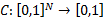where:

(a)    There are (uniform) random variables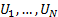taking values in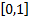such that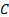is their cumulative (multivariate) distribution function; and

(b)has uniform marginal distributions, i.e. for all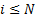and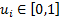we have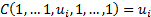The basic rationale for copulas is that any joint distribution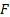of a set of random variables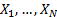i.e.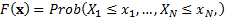can be separated into two parts. The first is the combination of the marginal distribution functions for each random variable in isolation, also called the marginals, i.e.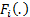where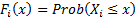. The second is the copula that describes the dependence structure between the random variables. Mathematically, this decomposition relies on Sklar’s theorem, which states that ifare random variables with marginal distribution functions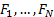and joint distribution functionthen there exists an N-dimensional copulasuch that for all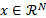: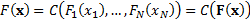Copulas can be of any dimension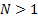. For example, the co-depency between 3 different series cannot be encapsulated merely by 3 different elements characterising the co-dependency between each pair of series. A quantum mechanical analogue would be the possibility that 3 different quantum mechanical objects can be ‘entangled’ in more complex ways than is possible merely by considering each pair in turn.

The simplest copulas are two-dimensional ones that describe aspects of the co-depencency merely between two different random variables. These are prototypical of more complicated copulas. Such a copula,, has the following properties:

(a)   Its domain,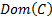, i.e. the range of values for which it is defined, is a unit square, i.e.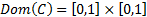(b)    It adheres to the following relationships: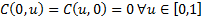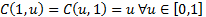(c)   It is ‘2-increasing’, i.e.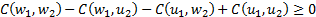whenever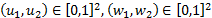and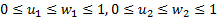The copula function of random variables is invariant under a strictly increasing transformation. Thus the copula completely encapsulates the dependence characteristics between different random variables.

Certain relationships and limits can be derived on the values that copulas can take. Fréchet copulas corresponding to the lower and upper bounds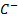and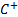are: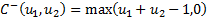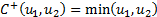Any two-dimensional copula,, satisfies the following ordering, which is called the concordance order (for distributions) or the stochastic order (for random variables)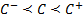Another important special case is the product (or independence) copula, see  which is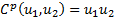.

Two random variables,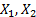are said to be:

(a)    countermonotonic if(b)    independent if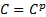(c)     comonotonic if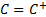Information on several of the above copulas (and on other probability distributions) is available here, including details of the following copulas: Clayton, Comonotonicity, Countermonotonicity, Frank, Generalised Clayton, Gumbel, Gaussian, Independence and t copulas.